# VCO using the TL431 reference

## TL431 TL431A TL431B KA431 LM431

R O Ocaya

EDN

The TL431 is a three-terminal programmable shunt regulator that implements Zener-like references with low temperature coefficients. Its output can be programmed from the internally set reference of about 2.5 V to 36 V using two external resistors. In addition, it exhibits a wide operating current range of 1.0 mA to 100 mA with a typical dynamic impedance of 0.22 Ω. The characteristics of these references make them excellent replacements for zener diodes in many applications such as digital voltmeters, power supplies, and op-amp circuitry, where precision voltage references are needed. Today, they are ubiquitous in switching-mode power supplies.The TL431 exhibits an interesting instability under certain conditions of input supply voltage and capacitive load, causing sustained oscillations that can range from 10 kHz up to 1.5 MHz, generally depending on the control input voltage. This occurs partly because of a negative resistance region under those conditions. As shown in this Design Idea, the instability neither arises from the presence of two internal poles, nor from a third pole introduced from an external capacitor in series with the load resistance (Figure 1). A single transistor output stage is added to provide buffering, producing a TTL output level over the entire range.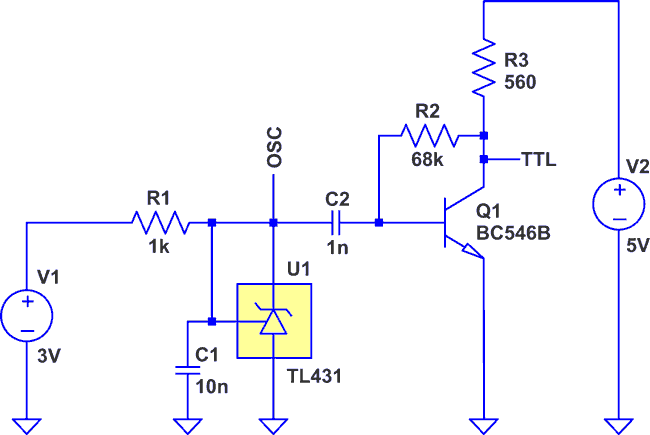Figure 1. Circuit of the TL431 VCO with output buffered to TTL levels.

Operation of the VCO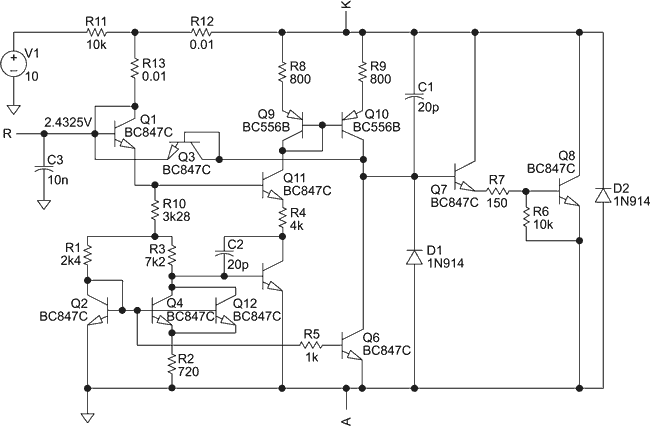Figure 2. TL431 equivalent circuit for LTSpice.

The operation of the oscillator can be understood by considering the circuit in two aspects. The first aspect is the underlying action of the TL431 voltage reference. Consider the equivalent circuit of the oscillator shown in Figure 2. Current I1 (see Figure 3) is a voltage-dependent constant current whose magnitude is approximately equal to

(VKA being the “Zener” voltage). Suppose initially that the capacitor is not charged, in which case VKA = 0 V. The capacitor gradually charges up from a current derived from I1 until it reaches the equilibrium value of the TL431, i.e., VKA = 2.49 V. Since charging current is still present, the capacitor continues to charge. A transient simulation of the circuit in Figure 2 shows that the capacitor voltage need only exceed the equilibrium value of VKA by a few microvolts for the equilibrium restoring feedback of the device to kick in, as follows: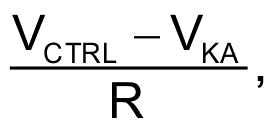As the base of Q1 is directly connected to the capacitor, an increase in VKA also increases the emitter voltage of Q1 (which is also the base voltage of Q11), forcing Q11 to conduct more. Transistor Q9 and R8 form the collector load of Q11. A rising collector current in Q11 therefore causes the collector voltage of Q9 to fall. Since Q9 and Q10 are part of a current mirror, the two transistors have the same collector current as Q11, but Q10 has a dynamic collector load made up of Q6, which derives its base current through R5 from the second current mirror made up of the three transistors Q2, Q4, and Q12. This current mirror is configured such that the initial increase in the emitter voltage of Q1 also increases their VBE voltage. This affects Q6 by also increasing its collector current, reinforcing the rising collector current in Q10. Therefore the overall effect is an increase in its collector voltage, which is also the base voltage of the first transistor in the Darlington pair Q7 and Q8, forcing Q8 to conduct heavily, causing its collector-emitter voltage (VCE) which in effect is VKA to fall rapidly. In this particular application the reference terminal (R) to which the capacitor is connected is hardwired to the cathode terminal (K). Therefore, thus far, when the capacitor voltage exceeds the equilibrium, the device tends to rapidly lower its cathode-anode voltage to restore equilibrium.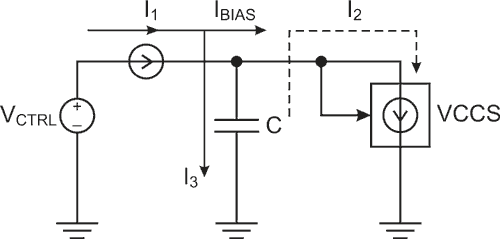Figure 3. Simple illustration of the current paths in the TL431 relaxation oscillator.

Figure 3 shows in block schematic form how sustained oscillations start and build up when the internal equilibrium of the TL431 device is disturbed. The capacitor charges from a small, almost constant current that is derived from supply current I1. In Figure 3, this charging current is denoted by I3. When the capacitor passes the equilibrium value of VREF, current I2, which is the combined collector currents of Q7 and Q8 in Figure 2, rapidly flows and effectively siphons off charge stored in the capacitor. The duration of I2 is brief, but is sufficient to drop the capacitor voltage below the equilibrium again. The capacitor then begins to charge from I1 again, and the cycle attains steady oscillation. Since the discharge of the capacitor occurs very briefly, the current during discharge is very much larger than source current I1, according to I = ΔQ/Δt, where ΔQ is the charge acquired by the capacitor during its charging phase.

Estimation of charging and discharging times

Since the charging and discharging currents are known, approximate expressions for the charge acquired during charging, and the charge dumped into the output stages of the TL431, can be found. The two expressions are equal during stable oscillation, a process akin to a two step bucket brigade. That is, charge acquired during charging equals charge lost during discharging.

In Figure 3,

I3 = I1 – IBIAS.

The magnitude of IBIAS in the TL431 is about 260 μA over a wide range of VCTRL.

From first principles, the following differential equation results: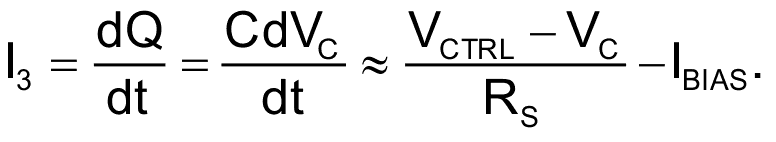The resistance RS is the series resistance connected to the control voltage. Solving the differential equation from the lower threshold to the upper threshold ( ) of VC during stable oscillation gives the charging time as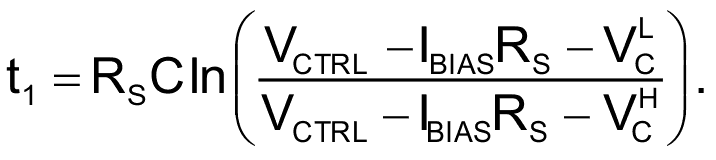The discharge time is slightly more complex to estimate because the discharge occurs through a dynamic resistance. The effective resistance through which the acquired charge is dumped during discharge can be estimated as follows. Simulations and experiments show that during stable oscillation, VKA does not fall below about 1.60 V or exceed about 2.74 V. An inspection of the TL431 datasheet, Figure 4, shows how, like a diode, the device exhibits dynamic resistance.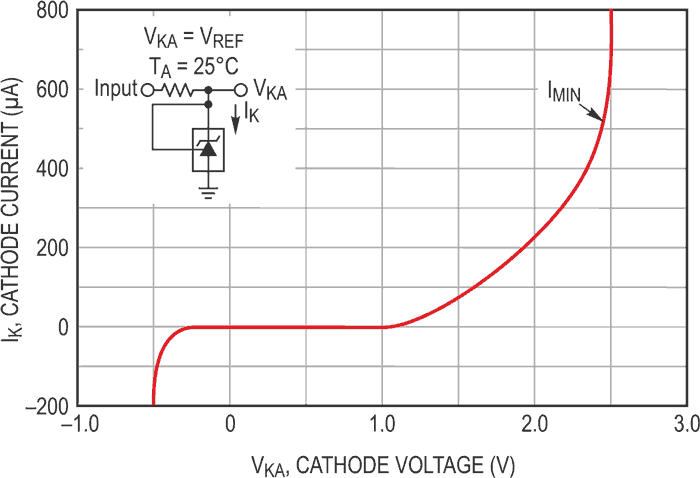Figure 4. Characteristic of the TL431B showing dynamic resistance. Reproduced from .

This is a diode-like forward bias characteristic that can therefore be approximated by the function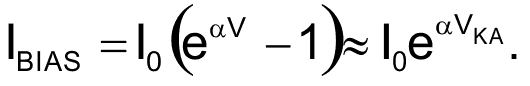Unlike normal junction diodes, the TL431 current has no significant temperature coefficient since it is designed around a band gap reference. The dynamic resistance can be shown to be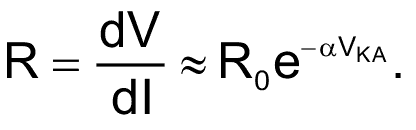A linear fit of the datasheet characteristic gave R0 ≈ 135.9 kΩ, α ≈ 2.304 V/kΩ. Hence, in the region of oscillatory behaviour, the resistance changes from 1.7 kΩ to 246 Ω. In the context of capacitive discharge this means that as the control voltage increases, the discharge occurs faster since the effective path for discharge has lower resistance. Therefore, one expects that the discharge time will decrease – i.e., frequency will increase as the control voltage increases. This is in fact observed in the practical oscillator. Simulation shows that the discharge involves a large current flowing from the capacitor and therefore the discharge time is typically very short and can be ignored.

The output of the oscillator is directly taken across the capacitor, and external buffering is therefore necessary to prevent loading the capacitor. Other LTSpice models of the TL431 are readily available from a variety of sources – for example, the Texas Instruments website, and the LTspice Wiki. The circuit in Figure 1 was simulated using the different models, and all of them oscillated consistently (Figure 5). In the practical experiments, several equivalents were used from different manufacturers. The TL431A and TL431B, the KA431, and the LM431 were all tried, and though all of them oscillated, the voltage input for oscillation onset and the range of frequency oscillation varied for each. In addition, the reference voltages of the devices were spread from 2.43 V to +2.53 V.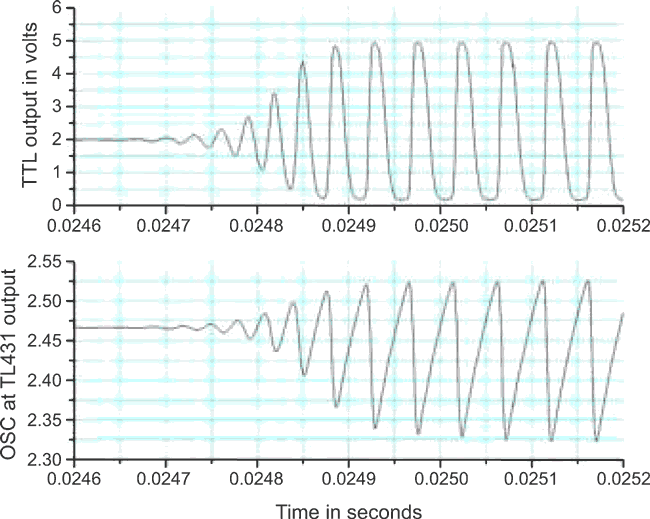Figure 5. Simulated outputs of the TL431 VCO.

The output amplitude of the oscillator at the point labelled OSC in Figure 1 is observed to vary as the control input V1 is varied, increasing as V1 increases. Under the currents used in the practical circuits it was observed that the frequency output is about proportional to the control voltage input, but at certain currents, measurements actually showed the same variation up to a certain control voltage (region 1), and then the frequency of oscillation fell as the control voltage is increased (region 2). For the linear region 1, Table 1 gives a sense of the component values and the frequency range versus control input for the different devices tested.

Table 1.
Practical VCO measurements on the TL431 in the region in which frequency
increases with control voltage
 Capacitance (C1) in nF KA431 LM431 Control voltage (V1) range in volts Output frequency range in kHz Control voltage (V1) range in volts Output frequency range in kHz 10 2.69 – 3.39 52.1 – 150.1 3.90 – 5.75 934.0 – 1517.0 100 2.69 – 11.6 12.4 – 211.5 7.47 – 11.60 383.0 – 435.0

In these experiments the control voltage was limited to about 12 V in the interest of limiting the cathode currents to 10 mA, although the TL431 datasheet states that the device can sink up to 100 mA. In addition, only the LM431 exhibited region 2, i.e., when the frequency actually decreased with increasing control voltage. This happened between 5.20 V and 7.04 V control input and a corresponding frequency range of 602 kHz to 433 kHz. The value for C1 was 100 nF. According to the datasheet , the range of capacitance for these instabilities to occur is 10 nF to 100 nF – the same range covered here.

Possible applications include an ultra-low cost laboratory TTL pulse generator and a low-frequency PLL VCO usable in the medium-wave band. The unit was successfully used in a diode-ring mixer circuit to realize a software defined radio (SDR) for the AM band.

References

1. Motorola Inc., TL431A,B Programmable precision references, Document TL431/D Revision 6, 1998 (Current TI datasheet)

Appendix

Video of the VCO in action